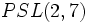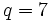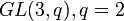# Projective special linear group:PSL(3,2)

Jump to: navigation, search
This article is about a particular group, i.e., a group unique upto isomorphism. View specific information (such as linear representation theory, subgroup structure) about this group
View a complete list of particular groups (this is a very huge list!)[SHOW MORE]

## Definition

This group is defined in many equivalent ways:

1. It is the projective special linear group of degree three over the field of two elements, i.e.,$PSL(3,2)$.
2. It is the special linear group of degree three over the field of two elements, i.e.,$SL(3,2)$.
3. It is the projective general linear group of degree three over the field of two elements, i.e.,$PGL(3,2)$.
4. It is the general linear group of degree three over the field of two elements, i.e.,$GL(3,2)$.
5. It is the projective special linear group of degree two over the field of seven elements, i.e.,$PSL(2,7)$.
6. It is the conformal automorphism group of the Klein quartic surface, which is a Riemann surface and in particular a Hurwitz surface. Hence, this group is a Hurwitz group, and is in fact the unique Hurwitz group of smallest order.

### Equivalence of definitions

The equivalence between definitions (1)-(4) follows from isomorphism between linear groups over field:F2.

## Arithmetic functions

Function Value Similar groups Explanation
order (number of elements, equivalently, cardinality or size of underlying set) 168 groups with same order As$\! GL(n,q), n = 3, q = 2$:$(q^n - 1)(q^n - q) \dots (q^n - q^{n-1}) = (2^3 - 1)(2^3 - 2)(2^3 - 2^2) = 7 \cdot 6 \cdot 4 = 168$
As$\! PSL(2,q), q = 7$:$\! (q^3 - q)/2 = q(q - 1)(q + 1)/2 = (7)(6)(8)/2 = 168$
exponent of a group 84 groups with same order and exponent of a group | groups with same exponent of a group Elements of order$2,3,4,7$.
derived length -- not a solvable group.
nilpotency class -- not a nilpotent group.
Frattini length 1 groups with same order and Frattini length | groups with same Frattini length Frattini-free group: intersection of maximal subgroups is trivial.
minimum size of generating set 2 groups with same order and minimum size of generating set | groups with same minimum size of generating set Generated by an element of order$2$ and an element of order$3$.
subgroup rank of a group 2 groups with same order and subgroup rank of a group | groups with same subgroup rank of a group --
max-length of a group 5 groups with same order and max-length of a group | groups with same max-length of a group

### Arithmetic functions of a counting nature

Function Value Explanation
number of subgroups 179
number of conjugacy classes 6 As$PSL(2,q)$,$q = 7$:$(q + 5)/2 = (7 + 5)/2 = 6$
As$GL(3,q), q = 2$:$q^3 - q = 2^3 - 2 = 6$
As$SL(3,q), q = 2$:$q^2 + q = 2^2 + 2 = 6$
number of conjugacy classes of subgroups 15

## Group properties

Property Satisfied Explanation
Abelian group No
Nilpotent group No
Metacyclic group No
Supersolvable group No
Solvable group No
Simple group Yes The second smallest simple non-abelian group (hence finite simple non-abelian group).
T-group Yes
HN-group No
Monolithic group Yes
One-headed group Yes
Hurwitz group Yes It is the conformal automorphism group of the Klein quartic surface, which has genus three, and its order is$84(3 - 1) = 168$.

## GAP implementation

### Group ID

This finite group has order 168 and has ID 42 among the groups of order 168 in GAP's SmallGroup library. For context, there are 57 groups of order 168. It can thus be defined using GAP's SmallGroup function as:

SmallGroup(168,42)

For instance, we can use the following assignment in GAP to create the group and name it$G$:

gap> G := SmallGroup(168,42);

Conversely, to check whether a given group$G$ is in fact the group we want, we can use GAP's IdGroup function:

IdGroup(G) = [168,42]

or just do:

IdGroup(G)

to have GAP output the group ID, that we can then compare to what we want.

### Other descriptions

Description Functions used
PSL(3,2) PSL
PGL(3,2) PGL
SL(3,2) SL
GL(3,2) GL
PSL(2,7) PSL
PSU(2,7) PSU